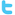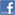Genesis Insoft The name you can TRUSTHome  |  Contact Us |  Careers
Java Question Bank / FAQs

This is a mock Exam based on the Objectives for the Sun Java Programmers Exam. It is created by Genesis InSoft Limited (admin@genesisinsoft.com) and may be freely distributed so long as it is unmodified. Please email us if you have any corrections or comments.

##### Question 1

Which of the following converts an array of bytes to an instance of class Class?

1. toClass( )
2. createClass()
3. byteToClass()
4. defineClass()
##### Question 2

What is the general form of declaration for a method in Java?

1. accessspecifier  modifier  returnvalue  name of method  (parameters)   throws  ExceptionList
2. modifier  accessspecifier  returnvalue  name of method  (parameters)   throws  ExceptionList
3. accessspecifier  returnvalue  modifier  name of method  (parameters)   throws  ExceptionList
4. modifier  returnvalue  accessspecifier  name of method  (parameters)   throws  ExceptionList
##### Question 3

What is the default Container layout combination  for a Panel?

1. FlowLayout
2. CardLayout
3. BorderLayout
4. GridLayout
##### Question 4

________ a thread group is automatically destroyed when its last thread is stopped or its last

1. Daemon
2. Auto
3. Default
4. none
##### Question 5

Which is the followig method returns the current number of active threads in this thread group

1. currentCount()
3. activeCount()
4. getActiveCount()
##### Question 6

What is the output of the following code?

int i = 16;
int j = 17;
System.out.println("i >> 1  =  " + (i >> 1));
System.out.println("j >> 1  =  " + (j >> 1));

1. Prints "i >> 1 = 8"
"j >> 1 = 8"
2. Prints "i >> 1 = 7"
"j >> 1 = 7"
3. Prints "i >> 1 = 8"
"j >> 1 = 9"
4. Prints "i >> 1 = 7"
"j >> 1 = 8"
##### Question 7

Which of the operators have the lowest precedence in Java Operators?

1. Parentheses i.e [ ], ()
2. Conditional i.e ?:
3. Unary operators i.e +, -, ++, --
4. Assignment Operator i.e =
##### Question 8

1.    public class Q11{
2.        static String str1 = "main method with String[] args";
3.        static String str2 = "main method with int[] args";
4.        public static void main(String[] args){
5.            System.out.println(str1);
6.        }
7.        public static void main(int[] args){
8.            System.out.println(str2);
9.        }
10.  }

What will happen if you compile/run the following code?

1. Duplicate method main(), compilation error at line 6.
2. Duplicate method main(), compilation error at line 11.
3. Prints "main method with main String[] args".
4. Prints "main method with main int[] args".
##### Question 9

class Th22{
public static void main(String args[ ]){
int i = -333;
int j = ~i;
System.out.println(j);
}
}

The output of the above program is

1. Compilation error . ~ operator applicable to boolean values only.
2. Prints 333.
3. Prints 332
4. Prints 334
##### Question 10

What is the output of the following code?

1.    class StringTest {
2.        public static void main(String args[ ]){
3.            String str = "Welcome";
4.            str.concat(" to Java!");
5.            System.out.println(str);
6.        }
7.    }

1. Strings are immutable, compilation error at line 3.
2. Strings are immutable, runtime exception at line 3.
3. Prints "Welcome".
4. Prints "Welcome to Java!".
##### Question 11

What types of files can be stored in JAR archives?

1. XML files
2. BMP files
3. Class files
4. All of the above
##### Question 12

What does the stop() internally do to stop a thread ?

1. it calls the destroy() method of the Thread class.
2. it calls the yield() method of the Thread class.
3. it throws an instance of the ThreadDeath class.
4. None of the above.
##### Question 13

What is the only method available in the Runnable inteface ?

1. start().
2. stop().
3. run().
4. sleep().
##### Question 14

What will happen if you compile/run the folowing lines of code?

Vector a = new Vector();
System.out.println(a.elementAt(0));

1. Prints 10.
2. Prints 11.
3. Compilation error.
4. Prints some garbage.
##### Question 15

What will happen if you invoke the following method?

public void check() {
System.out.println(Math.min(-0.0,+0.0));
System.out.println(Math.max(-0.0,+0.0));
System.out.println(Math.min(-0.0,+0.0) == Math.max(0.0,+0.0));
}

1. prints -0.0, +0.0 and false.
2. prints -0.0, +0.0 and true.
3. prints 0.0, 0.0 and false.
4. prints 0.0, 0.0 and true.
##### Question 16

public class Test {
//Some code comes here
}

Which of the following can be used to define a constructor for this class?

1. public void Test() {...}
2. public Test() {...}
3. public static Test() {...}
4. public static void Test() {...}
##### Question 17

What tags are mandatory when creating HTML to display an applet?

1. name, height, width.
2. code, name.
3. codebase, height, width.
4. code, height, width
##### Question 18

Characters in java are based on which encoding scheme ?

1. Unicode encoding scheme.
2. ASCII / ANSI.
3. UTF 8.
4. None of the above.
##### Question 19

public class MyFor{
public static void main(String argv[]){
int i; int j;
outer:for (i=1;i <3;i++){
inner:for(j=1; j<3; j++) {
if (j==2)
continue outer;
System.out.println("Value for i=" + i + " Value for j=" +j);
}
}
}
}

What is the output of the above program?

1. Value for i=1 value for j=1.
2. Value for i=2 value for j=3.
3. Value for i=2 value for j=2.
4. Value for i=3 value for j=1
##### Question 20

Which of the following technologies depend on the "Serialization" mechanism?

1. JiniTM technology.
2. JavaSpacesTM technology.
3. RMI.
4. All of the above